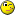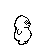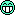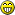# Determining Viscosity

#### MolaKule

Staff member
This exercise is a bit more involved and requires a Scientific Calculator and is open to all. One way of measuring viscosity is to use a "Rotating Concentric Cylinder" viscosimeter, sometimes called a, "Couette" viscosimeter. A rotating cylinder (the Inner Cylinder), is placed inside another (outer) cylinder and the fluid whose viscosity is to be measured in poured into the gap, g. The Inner Cylinder is rotated at a specific RPM and the amount of Torque to rotate the Inner Cylinder is measured. Note: * is multiplication and X^n is a number raised to the "nth" power since we don't have an equation editor in BITOG. The viscosity is given by: u = T*g/(4*pi^2*R^3*n*L) = Absolute Viscosity in SI units of N.s/m^2 T is torque, measured at 1.8 N.m, g is gap between Inner and Outer Cylinder, or 0.15 cm, Outer Diameter of Inner Cylinder is 12 cm, L is length of each each cylinder which is 40 cm long, n is RPM, 300 RPM R is Radius of Inner Cylinder. Hints: Use 39.48 for 4*pi^2; Convert all given units to meters and seconds; answer will be in N.s/m^2. We will disregard any "end" effects. The result will be a 30 Grade motor oil at ~ 40C.Last edited by a moderator:

#### Ohle_Manezzini

66.83 mm square per second.

#### MolaKule

Staff member
Hints: Use 39.48 for 4*pi^2; Convert all given units to meters and seconds; answer will be in N.s/m^2.

#### Garak

Showing units: 1.8 N.m × 0.0015 m / [4 × pi^2 × (0.06m)^3 × 5 rev/s × 0.4m] = 0.158314349 N.s/m^2 = 0.2 N.S/m^2, given one significant figure.Calculating through the units does indeed show the units you asked for are obtained.

Last edited:

#### Ohle_Manezzini

Force? Newton * second / square meters

#### Garak

Newtons are the force, this expression is a dynamic viscosity, where 1 N.s/m^2 = 10 P or 1000 cP.

#### MolaKule

Staff member
Originally Posted By: Ohle_Manezzini
Force? Newton * second / square meters
Excellent Garak.RPO, had I given you the density you could have determined the Kinematic Viscosity in the SI units of m^2/s or cgs units of centistokes or cSt, with proper conversion coefficients.

#### Ohle_Manezzini

So the answer can also be expressed as 200 cP at 40C... Or: In cst is 200 In Poise is 2 In stokes is 2 In SSU is 927 RPO

Last edited:

#### Garak

Originally Posted By: MolaKule
Excellent Garak.
Thanks. Technically, I shouldn't have put rev/s as a unit in my equation, since revs really aren't a unit. To be rigorous, I should have just used the unit of s^-1.#### Ohle_Manezzini

And the range for a 30 weight oil @ 40C is: 55cP to 82cP! So, this oil isn't a 30 grade at all Sorry Mola, could't resist, just grabbed a coffeeCqd,, RPO

#### MolaKule

Staff member
Originally Posted By: Ohle_Manezzini
And the range for a 30 weight oil @ 40C is: 55cP to 82cP! So, this oil isn't a 30 grade at all Sorry Mola, could't resist, just grabbed a coffeeCqd,, RPO
RPO, you need more than coffee.Look at F.M. White's, Fluid Mechanics, McGraw-Hill, 2003. He has a chart of various fluids (Viscosity vs. Temperature) expressed in Absolute Viscosity or N.s/m^2, and the SAE 30 oil [email protected] does indeed pass through the answer given by Garak.

#### Ohle_Manezzini

Ah, 20C, Ok,Staff member